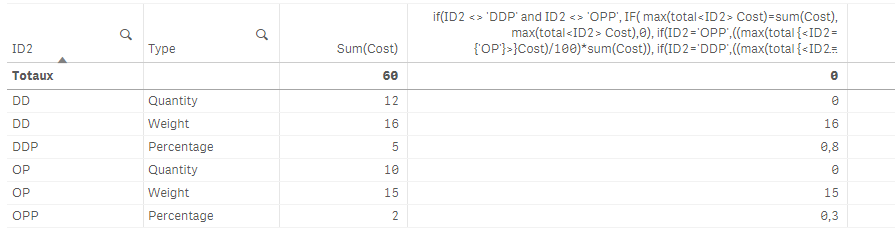# New to Qlik Sense

Discussion board where members can get started with Qlik Sense.

New Contributor

## Calculate Expression based on other row values

I have a scenario if one of the row value is percentage then I need to calculate the % of another row value. Explained below- I need a help to populate the last column.

ID             Type             Cost             Calculated Column

OP          Quantity          10               0

OP          Weight            15               15 (It have max cost of each ID.lower value will be replaced by 0)

DD          Quantity          12               0

DD          Weight            16               16

OPP        Percentage      2                 ? (2% of 15(max value of OP) )

DDP        Percentage       5                ?  ( 5% of 16(max value of DD) )

I have calculated max value by aggr function  =Sum(aggr(Max([Cost]),ID,Type)).

The above expression works good and max value calculation is done. However I couldn't get a logic to populate last two rows which is a percentage calculation.

If ID=OPP  and Type=Percentage it will have to calculate percentage of max value of OP (15 in this case).

If ID=DDP  and Type=Percentage it will have to calculate percentage of max value of DD (16 in this case).

I tried to achieve this by creating some intermediate columns as well but no luck so far. Any help would be much appreciated!

HighlightedPartner

## Re: Calculate Expression based on other row values

Try this expression:

if(ID <> 'DDP' and ID <> 'OPP', IF( max(total<ID> Cost)=sum(Cost), max(total<ID> Cost),0),

if(ID='OPP',((max(total {<ID={'OP'}>}Cost)/100)*sum(Cost)),

if(ID='DDP',((max(total {<ID={'DD'}>}Cost)/100)*sum(Cost))

)

)

)

result: